# Second Law Analysis for the Optimization of Plate Heat Exchangers

Second Law Analysis for the Optimization of Plate Heat Exchangers

Roberto BrunoPiero Bevilacqua Vittorio Ferraro Natale Arcuri

Mechanical Energetic and Management Engineering Department, University of Calabria, p. Bucci 46/c – 87036 – Arcavacata di Rende (CS), Italy

Computer Engineering, Modelling, Electronics and Systems Department, University of Calabria, p. Bucci 46/c – 87036 – Arcavacata di Rende (CS), Italy

Corresponding Author Email:
roberto.bruno@unical.it
Page:
475-482
|
DOI:
https://doi.org/10.18280/mmep.060401
20 October 2019
|
Accepted:
5 November 2019
|
Published:
24 December 2019
| Citation

OPEN ACCESS

Abstract:

The transfer of thermal power in reduced spaces can be attained by plate heat exchangers (PHE), making these devices suitable for different applications. The encumbrance is very limited due to the parallel plates installed with a reduced distance, so the fluids circulate in alternate manner inside the correspondent channel in a counter-current configuration. Appropriate corrugations on the plate surface are provided to improve the thermal transfer, however the frictions with the fluid can increase significantly. In this paper, a second law analysis involving the irreversibilities connected with the thermal transfer and pressure drops was carried out to identify the optimal PHE configuration by means of the minimal entropy generation approach. Because technical solutions employed to improve the thermal transfer among the fluids often determine a pressure drops growth, an optimal configuration can be identified. At this purpose, a quality dimensionless index related to the irreversibilities and determined with a parametric study by varying number of plates, pitch, corrugation angle, fluid flow rates and exchange surface material allowed to identify the optimized PHE.

Keywords:

design optimization, entropy generation minimization model, plate heat exchanger, parametric study

1. Introduction

The calculation of the entropy generation allows for the measurement of dissipated energy and of the degradation of the systems performance, therefore its minimization has been widely employed in the design phase to optimize several typologies of devices [1, 2]. Indeed, the energy transformation processes always determine a precise unavailable rate, and the remaining part decreases in every successive conversion, due to the process irreversibilities, reducing the energy “quality” . The aim of the approach based on the entropy generation minimization is to limit as much as possible the energy degeneration. The calculation of thermodynamic efficiency requires the knowledge of the energy that can be actually available, in accordance with the exergy concept; alternatively, the quantification of the generated irreversibility could be used. The latter is determined in function of the extensive property of a thermodynamic system denoted as “entropy”, whose concept was introduced by Carathéodory as a simple Pfaffian differential related to the multiplication factor T-1 , by validating the law of the entropy growth for real processes by means of the well-known inequality dS>0 . However, the entropy concepts assume a wider significance that extends beyond physical processes, evolving also in extra-physical systems [6-8]. By exploiting also the relation with the order and disorder concepts, the entropy was used to optimize urban planning process , or to develop Smart cities models .

A practical case corresponding to a physical process of great interest in thermodynamic science is represented by the thermal power transferred among fluids in heat exchangers . The optimization of these devices usually is attained with thermodynamic and/or economic criteria; alternatively the entropy generation minimization approach can be used to limit the irreversibilities associated with the involved physical processes, represented prevalently by the heat transfer among the fluids and the pressure drops inside the channels. Consequently, different heat exchanger configurations obtained by varying geometrical and thermal parameters produce different entropy generations, whose minimal value identify the optimized device design. This approach was widely employed in the scientific literature, involving different types of heat exchangers and different operation modality (co or counter current, shell and tubes, etc.) [12-15].

In this paper, plate heat exchangers (PHE) have been considered because largely employed in different fields due to their capability to transfer noticeable thermal powers despite the compact size. PHEs use parallel-corrugated plates as exchange surfaces, where the fluids flow rates circulate in alternate manner in counter-current configuration (Figure 1). PHEs are flexible devices allowing for a modification of the exchange surface; indeed they can be easily disassembled to remove or to add a certain number of plates. The employment of corrugations on the exchange surfaces facilitates the achievement of turbulent flows and consequently improves the thermal exchange, however it determines noticeable frictions that increase with the reduction of the plates distance (pitch). The latter can be modified by exploiting the deformable gaskets between the plates, constrained by an upper and a lower guide bar, which can be compressed allowing for a pitch regulation by acting on apposite tie bolts. The number of plates, as well as their distance, the size, the type of “chevron” angle that characterizes the corrugation, the employed materials as well as the operative conditions, represent the main parameters investigated for the device optimization.

## 1.jpgFigure 1. PHE with 3 passes for each fluid flow rate

2. Mathematical Model Description

The calculation of the irreversibilities connected to the thermal transfer and the pressure drops in a counter-current PHE are based on the mass and the energy conservation laws. In order to simplify the calculations, the following assumptions were made: steady state flows, negligible thermal losses between the heat exchanger and the surrounding, isothermal plates and constant fluids thermal properties, the latter evaluated at a fluid mean temperature. Considering the infinitesimal portion of heat exchanger shown in Figure 2, the irreversibility connected with the heat transfer process between the hot (h) and the cold (c) flow rates, separated by a corrugated plate with constant temperature T, can be written as:

$d\dot{S}(T)={{\dot{m}}_{c}}d{{s}_{c}}-\frac{d\dot{Q}}{T}+{{\dot{m}}_{h}}d{{s}_{h}}+\frac{d\dot{Q}}{T}={{\dot{m}}_{c}}d{{s}_{c}}+{{\dot{m}}_{h}}d{{s}_{h}}$      (1)

from which the well-known relation for heat exchangers is obtained:

$\dot{S}(T)={{\dot{m}}_{c}}\cdot {{c}_{p,c}}\cdot \ln \frac{{{T}_{c,out}}}{{{T}_{c,in}}}+{{\dot{m}}_{h}}\cdot {{c}_{p,h}}\cdot \ln \frac{{{T}_{h,out}}}{{{T}_{h,in}}}$       (2)

The irreversibilities due to the pressure drops for the two fluids with density $\rho$, circulating with a velocity v along the channel with length L, can be written as:

\begin{align} & \dot{S}(p)=\frac{{{{\dot{m}}}_{h}}\cdot {{f}_{h}}\cdot L\cdot v_{h}^{2}}{2\cdot {{D}_{eq,h}}}\cdot \left( \frac{1}{L}\int\limits_{0}^{L}{\frac{dy}{{{T}_{h}}}} \right)+\frac{{{{\dot{m}}}_{c}}\cdot {{f}_{c}}\cdot L\cdot v_{c}^{2}}{2\cdot {{D}_{eq,c}}}\cdot \left( \frac{1}{L}\int\limits_{0}^{L}{\frac{dy}{{{T}_{c}}}} \right)= \\ & =\frac{{{{\dot{m}}}_{h}}\cdot \Delta {{p}_{h}}\cdot {{F}_{h}}(NTU,\tau ,\omega )}{{{\rho }_{h}}\cdot {{T}_{h,in}}}+\frac{{{{\dot{m}}}_{c}}\cdot \Delta {{p}_{c}}\cdot {{F}_{c}}(NTU,\tau ,\omega )}{{{\rho }_{c}}\cdot {{T}_{h,in}}}\ \ \ \ \ \,\ \, \\ \end{align} (3)

where, Dp quantifies the total pressure drops affecting the hot and the cold flow rates in the correspondent channels, whereas F is a function that takes into account the solution of the integral inside the round brackets of Eq. (3). It represents the mean value along L of the inverse of the absolute fluid temperature, depending on the following dimensionless parameters (assuming cold fluid with the lowest thermal capacity):

$NTU=\frac{{{H}_{e}}\cdot A}{{{{\dot{m}}}_{c}}\cdot {{c}_{p,c}}}$

(He global heat transfer coefficient, A total exchange surface);

$\tau =\frac{{{T}_{c,in}}}{{{T}_{h,in}}}$

$\omega =\frac{{{{\dot{m}}}_{c}}\cdot {{c}_{p,c}}}{{{{\dot{m}}}_{h}}\cdot {{c}_{p,h}}}$

In particular, for an unbalanced exchanger ($\omega \neq 1$):

${{F}_{h}}=\frac{\left\{ 1-\omega \cdot e{{\ }^{\left[ NTU(\omega -1) \right]}} \right\}\cdot \left[ \ln ({{\sigma }_{h}})+NTU(\omega -1) \right]}{NTU(\omega -1)\cdot \left[ 1-\tau \cdot \omega \cdot {{e}^{\ \left[ NTU(\omega -1) \right]}} \right]}$            (4)

${{F}_{c}}=\frac{\left\{ 1-\omega \cdot e{{\ }^{\left[ NTU(\omega -1) \right]}} \right\}\cdot \left[ \ln ({{\sigma }_{c}})+NTU(\omega -1) \right]}{NTU(\omega -1)\cdot \left[ 1-\tau \cdot \omega \cdot e{{\ }^{\left[ NTU(\omega -1) \right]}} \right]}$                 (5)

with:

${{\sigma }_{h}}=\frac{1-\omega +\omega \cdot \tau \cdot \left\{ 1-e{{\ }^{\left[ NTU\cdot (\omega -1) \right]}} \right\}}{1-\omega \cdot {{e}^{\ \left[ NTU\cdot (\omega -1) \right]}}}$                    (6)

${{\sigma }_{c}}=\frac{\tau \cdot \left\{ 1-\omega \cdot e{{\ }^{\left[ NTU\cdot (\omega -1) \right]}} \right\}}{1-e{{\ }^{\left[ NTU\cdot (\omega -1) \right]}}-\tau \cdot (\omega -1)\cdot e{{\ }^{\left[ NTU\cdot (\omega -1) \right]}}}$                    (7)

## 2.jpg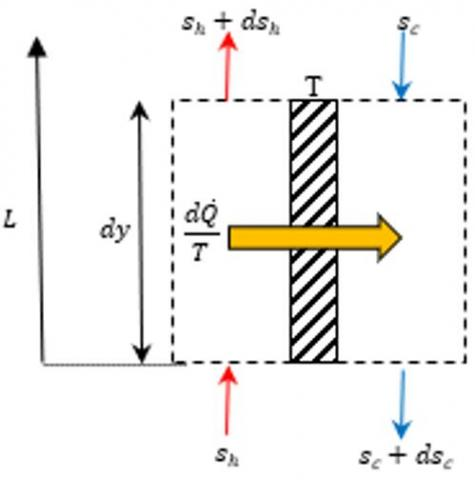Figure 2. Entropic balance for a PHE configuration in counter-current operating

Regarding the geometrical parameters of Figure 3, the pressure drops for each fluid (in Pa) can be determined by the following relation :

$\Delta p=4\cdot f\cdot \left( \frac{{{L}_{eff}}\cdot N}{{{D}_{eq}}} \right)\cdot \left( \frac{G_{c}^{2}}{2{{\rho }_{av}}} \right)\cdot {{\left( \frac{{{\mu }_{av}}}{{{\mu }_{W}}} \right)}^{0.17}}+1.4\cdot N\cdot \left( \frac{G_{p}^{2}}{2{{\rho }_{av}}} \right)$        (8)

where, the hydraulic diameter Deq can be set equal to 2 times the pitch b between the plates , Gc is the fluid flow rate normalized on the channel section area (b∙w), Gp the fluid flow rate normalized to the port area (0.25∙p∙Dp²) and N the number of passes.

The friction factor f for corrugated plates is function of the “chevron” angle $\beta$ with the following correlation :

$f={{\left( \frac{\beta }{30} \right)}^{0.83}}\cdot {{\left[ {{\left( \frac{30.2}{\operatorname{Re}} \right)}^{5}}+{{\left( \frac{6.28}{{{\operatorname{Re}}^{0.5}}} \right)}^{5}} \right]}^{0.2}}$               (9)

## 3.jpgFigure 3. PHE main geometrical parameters

where, the Reynolds number is determined in function of the hydraulic diameter and the fluid velocity, by calculating its density and viscosity at the average temperature along the PHE channel with length L. These temperatures can be determined with the following relations for the hot and the cold flow rate respectively (in counter-current configuration):

${{\bar{T}}_{h}}=\frac{\left[ \left( \frac{\omega }{\omega -1} \right)\left( {{T}_{h,in}}-{{T}_{c,out}} \right)\left( 1-{{e}^{\frac{{{H}_{e}}\cdot A\cdot (1-\omega )}{\omega \cdot {{{\dot{m}}}_{h}}\cdot {{c}_{p,h}}}}} \right)+\frac{{{H}_{e}}A\left( \omega {{T}_{c,out}}-{{T}_{h,in}} \right)}{\omega \cdot {{{\dot{m}}}_{h}}\cdot {{c}_{p,h}}} \right]}{\frac{(\omega -1)\cdot {{H}_{e}}\cdot A}{\omega \cdot {{{\dot{m}}}_{h}}\cdot {{c}_{p,h}}}}$                 (10)

${{\bar{T}}_{c}}=\frac{\left[ \left( \frac{1}{\omega -1} \right)\left( {{T}_{h,in}}-{{T}_{c,out}} \right)\left( 1-{{e}^{\frac{{{H}_{e}}\cdot A\cdot (1-\omega )}{\omega \cdot {{{\dot{m}}}_{h}}\cdot {{c}_{p,h}}}}} \right)+\frac{{{H}_{e}}A\left( \omega {{\cdot }_{c,out}}-{{T}_{h,in}} \right)}{\omega \cdot {{{\dot{m}}}_{h}}\cdot {{c}_{p,h}}} \right]}{\frac{(\omega -1)\cdot {{H}_{e}}\cdot A}{\omega \cdot {{{\dot{m}}}_{h}}\cdot {{c}_{p,h}}}}$                  (11)

The arithmetic media of the values provided by Eq. (10) and (11) is assumed as the average temperature of the isothermal plate required for the calculation of the viscosity $\mu_{W}$ appearing in Eq. (8). The global heat transfer coefficient is determined in function of the convective heat transfer coefficients of the fluids, determined with the thermo-physical properties at the film temperature calculated as mean value between the fluid and the wall average temperatures, and the thickness of the material constituting the plate. Analytically:

${{H}_{e}}={{\left( \frac{1}{{{{\bar{h}}}_{h}}}+\frac{1}{{{{\bar{h}}}_{c}}}+\frac{t}{{{\lambda }_{W}}} \right)}^{-1}}$                         (12)

where, $\bar{h}$ is the mean convective heat transfer coefficient determined both for the cold and the hot fluid, t is the plate thickness and $\lambda_{\mathrm{W}}$ its thermal conductivity. The firsts have been calculated with the following correlations , for turbulent flows (due to the presence of the corrugations):

$Nu=\frac{\bar{h}\cdot {{D}_{eq}}}{{{\lambda }_{av}}}=C(\beta )\cdot {{\operatorname{Re}}^{n(\beta )}}\cdot {{\Pr }^{{}^{1}/{}_{3}}}\cdot {{\left( \frac{{{\mu }_{av}}}{{{\mu }_{W}}} \right)}^{0.14}}$                 (13)

where,

$C(\beta )=0.2668-6.967\cdot {{10}^{-4}}\beta +7.244\cdot {{10}^{-5}}{{\beta }^{2}}$                 (14)

$n(\beta )=0.728+0.0543\cdot \sin \left[ 3.7+\frac{2\pi \beta }{90} \right]$                (15)

Finally, the generated entropies connected with the thermal transfer and the pressure drops have been made dimensionless by the multiplicative factor $T_{c, i n} / \dot{Q}$.

By combining all the involved parameters, the dimensionless generated entropies can be calculated in compact manner by the following relations by introducing the heat exchanger efficiency $\varepsilon$:

EG(\Delta T)=\frac{\dot{S}(\Delta T)}{{\dot{Q}}}\cdot {{T}_{c,in}}=\left[ \frac{1}{\varepsilon \cdot \left( \frac{1}{\tau }-1 \right)} \right]\cdot \left\{ \begin{align} & \ln \left[ 1+\varepsilon \cdot \left( \frac{1}{\tau }-1 \right) \right]+ \\ & +\frac{1}{\omega }\cdot \ln \left[ 1-\omega \cdot \varepsilon \cdot (1-\tau ) \right] \\ \end{align} \right\}                   (16)

\begin{align} & EG(\Delta p)=\frac{\dot{S}(\Delta p)}{{\dot{Q}}}\cdot {{T}_{c,in}}=\left( \frac{\Delta {{p}_{h}}\cdot \tau }{{{\rho }_{h}}\cdot {{c}_{p,h}}\cdot \varepsilon \cdot \omega (1-\tau ){{T}_{h,in}}} \right)\cdot \\ & \left( {{F}_{h}}+\frac{\omega \cdot \Delta {{p}_{c}}\cdot {{\rho }_{h}}\cdot {{c}_{p,h}}}{\Delta {{p}_{h}}\cdot {{\rho }_{c}}\cdot {{c}_{p,c}}}\cdot {{F}_{c}} \right) \\ \end{align}                   (17)

In order to determine the best PHE configuration, the following dimensionless quality index has to assume the greatest value:

$\eta (S)=1-EG(\Delta T)-EG(\Delta p)$                              (18)

In the ideal case, a unitary value is attained due to the lacking of irreversibilities; conversely it tends to decrease with the entropy generation growths.

3. Methodology

A commercial PHE actually available on the market for the production of domestic hot water in boilers was considered for the analysis. This device is constituted by corrugated plates in stainless steel ($\lambda_{W}$= 27 W∙m-1∙K-1) with a width w=20 cm and a height Leff = 38 cm, for an effective exchange surface equal to 0.042 m² each. The corrugation V type is characterized by a chevron angle $\beta=60^{\circ}$ on the plate surfaces that have a mean thickness t of 1 mm. The connection with the hydraulic circuits occurs by four ports with diameter Dp of 1.25 inches to carry water mass flow rates set to 0.161 kg/s for the hot fluid entering at 70°C, and to 0.138 kg/s for the cold one whose inlet temperature is assumed equal and constant to 15°C. This situation represents a typical case of domestic hot water production starting from the hot fluid produced by a boiler. Assuming initially a constant water specific heat (4186 J∙kg-1∙K-1) and the declared heat transfer coefficient provided by the manufacturer He, for a precise number of plates (and consequently of passes) these data allow for the calculation of initial values of NTU and $\omega$, consequently of the PHE efficiency e  and of the transferred heat power $\dot{Q}$. Successively the latter allows for the calculation of the outlet temperatures and consequently of the mean fluid temperatures inside the channels ($\bar{T}_{h}$ and $\bar{T}_{c}$). So, the correspondent thermal properties are attained in order to estimate new convective heat transfer coefficients and a new He value. This approach is repeated iteratively until convergence is reached for He. Often, four iterations stabilize the results, thus the PHE quality index h(S) was calculated for the different configurations.

4. Results

In Figure 4, the trend of the entropic index is shown in function of the pitch b and of the number of plates N+1 by setting the other geometrical parameters to the mentioned values. Results show that the lowest pitch value of 1 mm produces a maximum quality index in proximity of 13 plates (namely for an exchange surface of 0.409 m²), successively it decreases due to the pressure drops irreversibility growth that prevails on the reduction of the entropy generated by the heat transfer. For a pitch of 1.5 mm, a maximum value can be still detected; this time for an exchange surface corresponding to 21 plates. Successively asymptotical trends were detected over 27 plates for greater pitch values. The dimensionless quality index tends to increase with the number of plates and the pitch, due to the prevailing reduction of the heat transfer irreversibilities rather than the pressure drops increase. It is interesting to note that reduced pitches lead to better quality indexes for limited exchange surfaces, whereas large pitches become preferable with the augment of the plates number. Consequently, a pitch of 1 mm offers better scores than a pitch of 3 mm when the number of plates is lower than 13, whereas better results can be achieved when the plates are greater than 27. Therefore, in order to exploit also limited encumbrances, at parity of plates number and in presence of limited exchange surface, low pitches result preferable.

At parity of exchange surface, an optimal pitch value can be identified, beyond which the entropic index tends to decrease slightly despite the reduction of pressure drops due to the augment of the heat transfer entropy (Figure 5). In particular, the effect connected to the temperature difference growth between the fluids determines an augment of the heat transfer irreversibilities that prevails on the reduction of the pressure drops entropy. For instance, by setting a number of plates equal to 25 (about 1 m²), the best score is determined with a pitch of 2.5 mm, whereas passing to 35 plates, (about 1.5 m²), the higher quality index is attained with a pitch of 4.5 mm.

Again, the score decreases with the pitch growth in presence of reduced exchange surfaces, whereas it increases rapidly with reduced pitches when the exchange area increases. Accordingly, when the exchange surface is set, the plates pitch can be regulated to attain the highest quality index.

For the considered PHE, the devices equipped with 51 plates distanced of 4.5 mm, whose quality index of 0.9875 is the highest score, represents the best configuration.

## 4.jpgFigure 4. Quality indexes in function of number of plates for different pitch values

## 5.jpgFigure 5. Quality indexes in function of the pitch for different exchange areas

Another investigated parameter is the “chevron” angle $\beta$ that was set to 30°, 45° and 60°; in particular the effects connected with the chevron angle where studied for two values of the pitch b. In Figure 6, the trends of the quality index in function of the number of plates and for the three considered chevron angles, by setting the pitch of 4.5 mm, are shown. Clearly, the employment of wide angles produces better scores, and the latter increase with the exchange surface growth. A more marked difference appears between 30° and the other two angles, however for large exchange surfaces not evident deviances between 45° and 60°, were detected. The augment of the chevron angle determines an increment of the friction factor f of Eq. (9) and consequently of the pressure drops and the correspondent irreversibilities. However, the same angle growth produces an improvement of the heat transfer coefficients for both the fluids, therefore He rises as well as the generated entropy, the simultaneous augment of the transferred thermal power determines a reduction of the dimensionless entropy EG. If the pitch value is decreased to 1 mm (Figure 7), a different trend was detected: firstly, a small dependence on the chevron angle can be observed, successively the quality indexes tend to reduce with the exchange surface growth.

Wider chevron angles are suggested in presence of limited plates number, conversely $\beta=30^{\circ}$ becomes preferable for large exchange surfaces. Therefore, when pitches are limited, the effect of the pressure drops growth prevails on the improvement of the thermal exchange between the fluids. Conversely, the better heat transfer coefficients produced by wider chevron angle provide better scores in presence of a limited number of plates. Furthermore, for 18 plates distanced by 1 mm, the chevron angle does not affect the entropy generation in evident manner.

## 6.jpg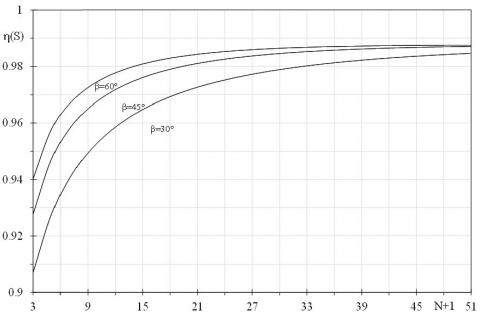Figure 6. Quality indexes in function of the chevron angle with a pitch of 4.5 mm

## 7.jpgFigure 7. Quality indexes in function of the chevron angle with a pitch of 1 mm

## 8.jpg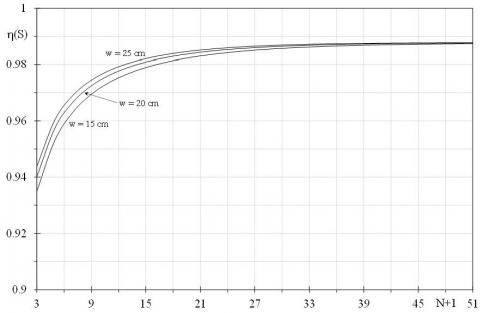Figure 8. Quality indexes varying the plate width with $\beta=60^{\circ}$ and pitch of 4.5 mm

In order to produce a variation of the exchange surface and of the passage section for both the fluids, the plate width w was varied at parity of height. In particular, other two widths were considered beyond the reference value: 15 cm and 25 cm. In Figure 8, the quality index trends for a PHE with a chevron angle of 60° and a pitch of 4.5 mm, is shown in function of the number of plates varying the width values. Clearly, the exchange surface growth leads to the improvement of heat transfer and the reduction of the pressure drops, consequently the quality index tends to increase. However, for a number of plates over 30, slightly variations have been detected. By reducing the pitch to 1 mm as depicted in Figure 9, similar trends can be observed but greater deviation between the considered widths can be observed. In particular, the largest width produces the better scores, however over a certain number of plates the dimensionless entropic indexes decrease due to the entropy generation connected to the pressure drops growth.

## 9.jpgFigure 9. Quality indexes varying the plate width with $\beta=60^{\circ}$ and pitch of 1 mm

## 10.jpg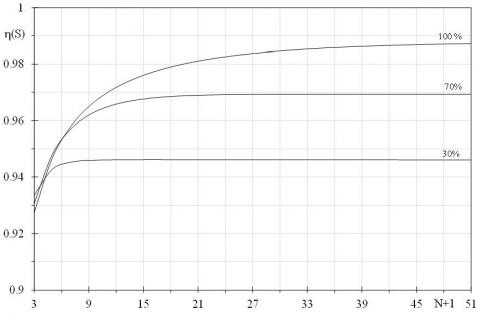Figure 10. Quality indexes varying the cold flow rate with $\beta=60^{\circ}$ and pitch of 7 mm

In Figure 10 the effects connected to the variation of the fluid flow rate are depicted; in particular, the cold flow rate was varied from the 30% to the 70% of the nominal value of 0.138 kg/s, by setting a wider pitch of 7 mm and $\beta=60^{\circ}$. Clearly, the flow rate reduction produces an evident decrement of the quality indexes due to the thermal transfer irreversibilities growth. Indeed, limited flow rates provides greater temperature difference between the inlet and the outlet, consequently the entropy generation connected to the heat transfer increases. It is interesting to highlight that slight improvements can be attained with the flow rate decrement in presence of limited exchange surfaces. Conversely, elevated flow rates produce better scores for high number of plates and an asymptotical trend is obtained beyond a precise number of plates, for every considered flow rate. Thus, for the investigated pitch, 6 plates should not be overcome when the cold flow rate is 30% of the nominal value, whereas 21 plates is the recommended number with the 70% of the nominal flow rate. Different trends were obtained by reducing the plates pitch to 1 mm and setting $\beta=60^{\circ}$, (Figure 11): this time, the lower is the flow rate, the smaller score is detected for every exchange surface. An asymptotical trend is not determined and a linear tendency was provided by setting the lowest cold flow rate value. In every case, it is confirmed the goodness to employ reduced exchange surface when the plate pitch is limited, also with a flow rate modulation.

Finally, in Figure 12 the trends obtained by varying the plate material, are shown.

## 11.jpg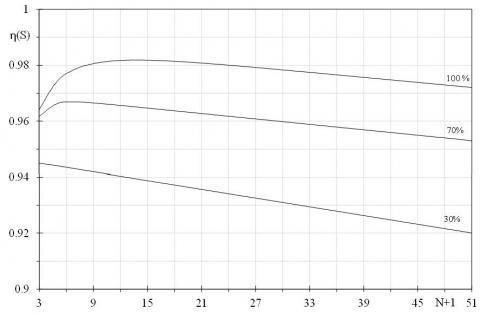Figure 11. Quality indexes varying the cold flow rate with $\beta=60^{\circ}$ and pitch of 1 mm

## 12.jpgFigure 12. Quality indexes with two different plate materials with $\beta=60^{\circ}$ and pitch of 4.5 mm

In particular, a comparison between a stainless steel plate and a copper plate ($\lambda_{W}$=340 W∙m-1∙K-1) is reported by setting a pitch of 4.5 mm and a corrugation angle $\beta=60^{\circ}$. Clearly, the advantage due to the higher thermal conductivity of the copper is evident in presence of limited exchange surfaces, whereas for an elevated number of plates the deviances between the quality indexes of the two materials are slightly different. Consequently, the employment of an expensive material as copper is justified only in presence of limited exchange surfaces.

5. Conclusions

The entropy generation minimization method was employed in order to determine the best configuration of a Plate Heat Exchanger (PHE) by varying the main geometrical features and the plate material. Considering a commercial PHE, a quality dimensionless index related to the irreversibilities due to heat transfer and pressure drops processes, was proposed. A parametric study carried out by varying the distance between the plates, their surface area, the cold fluid flow rate, the plate material and the chevron angle characterizing the plate corrugations, has allowed to identify PHE optimized configurations. The corrugations, in fact, are employed to increase the exchange surface and the thermal transfer between the fluids. However, these are responsible of noticeable frictions between the fluids and the plates, therefore irreversibilities connected to pressure drops cannot be neglected.

Moreover, the variation of several parameters affect in opposite manner the entropies due to the heat transfer process and the pressure drops, consequently an optimal balance point can be determined to identify the best PHE configuration. The considered irreversibilities were made dimensionless by normalizing with the actual transferred thermal power and the inlet temperature of the cold fluid, in order to determine a quality index as the complement of the total generated entropy. When the latter assumes the highest value, the best PHE configuration is recognized. In the ideal case, the quality index assumes a unitary value due to the absence of irreversibilities.

The quality index trends have shown that in correspondence of limited pitches, the effects connected to the pressure drop irreversibilities prevails on the augment of the exchange surface. Conversely, these pitch values allow for the attainment of better scores when the exchange surface is limited due to the improved thermal exchange that prevails on the pressure drops irreversibility. By setting the exchange surface, an optimal pitch can be identified for every PHE configuration and the score tends to decrease slightly with the pitch growth due to the greater temperature difference between the fluids that determines an increment of the heat transfer entropy, whereas irreversibilities connected with the pressure drops reduce.

In presence of high pitch values, the chevron angle should be set to wider values because it improves largely the heat transfer process, despite an increment of the pressure drops. For reduces pitches a wide chevron angle produces better score only for reduced exchange surfaces, otherwise reduced angle becomes preferable.

Finally, the augment of the exchange surface, at parity of the other investigated parameters, always leads to better scores, however asymptotical trends were detected for large pitches, consequently a limit value of the exchange area can be identified. Conversely, for reduced pitches an optimal exchange area can be found and it varies in function of the plate width. Globally, in presence of large exchange surfaces, the employment of high pitches, as well as of wider chevron angle and plates width, is always recommended. Instead, for reduced exchange areas, limited pitches produce better results, however wider chevron angles and plate widths should to be employed.

Finally, the employment of more conductive and expensive plate materials, as copper, is justified only in presence of limited exchange surfaces.

In conclusion, every PHE could be characterized by different optimal configurations in relation to the operating conditions, in particular the fluid flow rates that modify the heat transfer and the friction losses. However, the proposed model is very flexible allowing for the evaluation of the highest quality index that identifies the best PHE shape and size for different functioning conditions.

Nomenclature

A                exchange surface m²

b                plate pitch mm

cp               specific heat J∙kg-1∙K-1

C                Nusselt correlation coefficient

D                diameter m

EG             generated entropy

F                pressure drop function

f                 friction factor

G                normalized flow rate kg∙s-1∙m-2

$\bar{h}$                 mean convect. heat transf. coeff. W∙m-2∙K-1

He              PHE global heat transf. coeff. W∙m-2∙K-1

L                heat exchanger length m

$\dot{m}$                mass flow rate kg∙s-1

n                Nusselt exponent correlation

N                number of passes

NTU          PHE number of transfer units

Nu             Nusselt number

p                Pressure Pa

Pr               Prandtl number

$\dot{Q}$                 thermal power W

Re              Reynolds number

$\dot{S}$                 entropy W∙K-1

s                 specific entropy J∙kg-1∙K-1

t                 plate thickness mm

T                temperature K

v                fluid velocity m∙s-1

w                plate width cm

y                vertical direction

Greek symbols

$\beta$                chevron angle

$\Delta$                variation

$\varepsilon$                 PHE efficiency

$\lambda$                thermal conductivity W∙m-1∙K-1

$\mu$                dynamic viscosity Pa∙s

$\eta$                quality index

$\rho$              density kg∙m-3

$\sigma$                pressure drop coefficient

$\tau$                 temperature ratio

$\omega$                thermal capacity ratio

Subscripts

av              average

c                 cold

eff             plate height

eq              equivalent

h                hot

in               inlet

out             outlet

p                pipe port

w                wall

References

 Bruno, R., Bevilacqua, P., Ferraro, V., Arcuri, N. (2019). Entropy generation minimization for the design of plate heat exchangers. Tecnica Italiana – Italian Journal of Engineering Science, 63(2-4): 270-276. https://doi.org/10.18280/ti-ijes.632-422

 Bejan, A. (1995).  Entropy Generation Minimization: The method of thermodynamic optimization of finite-size systems and finite-time processes. First edition, CRC Press, Boca Raton (USA). https://doi.org/10.1201/9781482239171

 Prigogine, I. (1965).  Introduction to Thermodynamics of Irreversible Processes. Wiley, New York. https://doi.org/10.1149/1.2425756

 Carathèodory, C. (1909). Untersuchungen über die grundlagen der thermodynamik. Mathematische Annalen, 67: 355-386. https://doi.org/10.1007/BF01450409

 Sychev, V.V. (1991). The Differential Equations of Thermodynamics.  2nd edition, CRC Press.

 Nicoletti, G., Arcuri, N., Bruno, R., Nicoletti, G. (2016). On the generalized concept of entropy for physical, extra-physical and chemical processes. International Journal of Heat and Technology, 33(4): 21-38. https://doi.org/10.18280/ijht.34S103

 Martyushev, L.M., Seleznev, V.D. (2006). Maximum entropy production principle in physics, chemistry and biology. Physics Reports, 426: 1-45. https://doi.org/10.1016/j.physrep.2005.12.001

 Longo, G. (1980). Teoria dell'informazione. Boringhieri Edition, Turin (Italy).

 Arcidiacono, G., Arcidiacono, S. (2006). Entropia, sintropia, informazione. Una nuova teoria unitaria della fisica, chimica e biologia. 2nd Edition, Ed. Arcobaleno, Rome (Italy).

 Sguglio, A., Arcuri, N., Bruno, R. (2018). Integration of social science in engineering research for smart cities. The Italian Case of the RES NOVAE Project. Proceeding of 2018 IEEE International Conference on Environment and Electrical Engineering, Palermo (Italy). https://doi.org/10.1109/EEEIC.2018.8493774

 Bejan, A. (1977). The concept of irreversibility in heat exchanger design: counter flow heat exchangers for gas-to-gas applications. Journal of Heat Transfer, 99(3): 374-380. https://doi.org/10.1115/1.3450705

 Lerou, P.P.P.M., Veenstra, T.T., Burger, J.F., ter Brake, H.J.M., Rogalla, H. (2005). Optimization of counterflow heat exchanger geometry through minimization of entropy generation. Cryogenic, 45: 659-669. https://doi.org/10.1016/j.cryogenics.2005.08.002

 Johannessen, E., Nummedal, L., Kjelstrup, S. (2002). Minimizing the entropy production in heat exchange. International Journal of Heat and Mass Transfer, 45: 2649-2654. https://doi.org/10.1016/S0017-9310(01)00362-3

 Guo, J., Cheng, L., Xu, M. (2009). Optimization design of shell-and-tube heat exchanger by entropy generation minimization and genetic algorithm. Applied Thermal Engineering, 29(14-15): 2954-2960. https://doi.org/10.1016/j.applthermaleng.2009.03.011

 Ogulata, R.T., Doba, F. (1998). Experiments and entropy generation minimization analysis of a cross-flow heat exchanger. International Journal of Heat and Mass Transfer, 41(2): 373-381. https://doi.org/10.1016/S0017-9310(97)00129-4

 Neagu, A.A., Koncsag, C.I., Barbulkescu, A., Botez, E. (2016). Estimation of pressure drop in gasket plate heat exchangers. Ovidius University Annals of Chemistry, 27(1): 62-72. https://doi.org/10.1515/auoc-2016-0011

 Muley, A., Manglik, R.M. (1999). Experimental study of turbulent flow heat transfer and pressure drop in a plate heat exchanger with chevron plates. ASME Journal of Heat Transfer, 121(1): 110-117. https://doi.org/10.1115/1.2825923

 Mulley, A., Manglik, R.M. (2000). Enhanced thermal-hydraulic performance optimization of chevron plate heat exchangers. International Journal of Heat Exchangers, 1: 3-18.